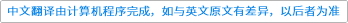Communications in Contemporary Mathematics ( IF 1.278 ) Pub Date : 2020-11-30 , DOI: 10.1142/s0219199720500790
Zaili Yan; Shaoqiang Deng

A quadruple of Lie groups $(G,L,K,H)$, where $G$ is a compact semisimple Lie group, $H⊂K⊂L$ are closed subgroups of $G$, and the related Casimir constants satisfy certain appropriate conditions, is called a basic quadruple. A basic quadruple is called Einstein if the Killing form metrics on the coset spaces $G/H$, $G/K$ and $G/L$ are all Einstein. In this paper, we first give a complete classification of the Einstein basic quadruples. We then show that, except for very few exceptions, given any quadruple $(G,L,K,H)$ in our list, we can produce new non-naturally reductive Einstein metrics on the coset space $G/H$, by scaling the Killing form metrics along the complement of $𝔥$ in $𝔨$ and along the complement of $𝔨$ in $𝔩$. We also show that on some compact semisimple Lie groups, there exist a large number of left invariant non-naturally reductive Einstein metrics which are not product metrics. This discloses a new interesting phenomenon which has not been described in the literature.down
wechat
bug